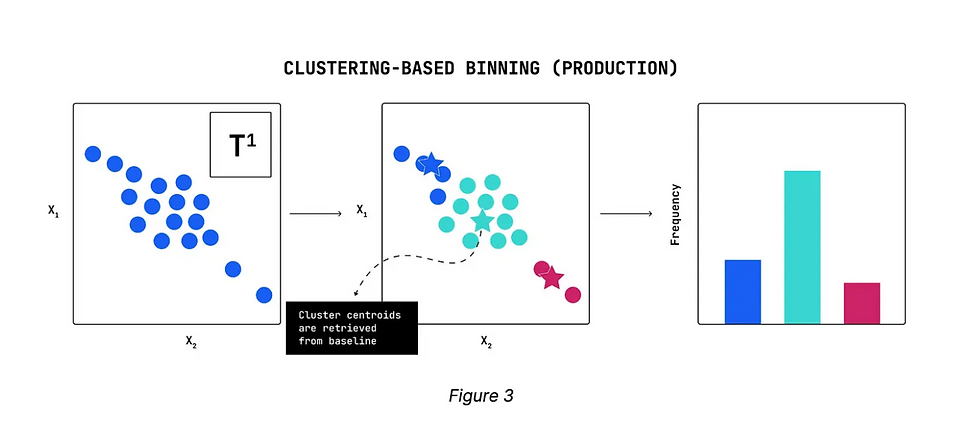top of page
Search

# Model Monitoring for Unstructured Data

A growing number of organizations build natural language processing (NLP) and computer vision (CV) models that are comprised of unstructured data, including text and images. These types of models support product and service innovation, as well as simplifying business operations.

However, common performance and drift monitoring methods, such as estimating univariate distributions using binned histograms and applying standard distributional distance metrics (e.g. Jensen-Shannon divergence) are not directly applicable to the case of high-dimensional vectors that represent unstructured data. The binning procedure in high-dimensional spaces is a challenging problem whose complexity grows exponentially with the number of dimensions. Furthermore, organizations gain actionable insights and context by detecting distributional shifts of high-dimensional vectors as a whole, rather than marginal shifts in vector elements.

It is important for ML teams to use a cluster-based drift detection method for monitoring high- dimensional vectors in order to precisely monitor drifts in unstructured models. In using such a method, ML teams can detect regions of high density (clusters) in the data space, and track how the relative density of such regions might change at production time.

In a cluster-based drift detection method, bins are defined as regions of high-density in the data space. The density-based bins are automatically detected using standard clustering algorithms such as K-means clustering. Once the histogram bins are achieved for both baseline and production data, then any of the distributional distance metrics can be applied for measuring the discrepancy between two histograms.

Figure 1 shows an example where the vector data points are 2-dimensional. Comparing the baseline data (left plot) with the example production data (right plot), there is a shift in the data distribution where more data points are located around the center of the plot. Note that in practice the vector dimensions are usually much larger than 2 and such a visual diagnosis is impossible.The first step of the clustering-based drift detection algorithm is to detect regions of high density (data clusters) in the baseline data. This is achieved by taking all the baseline vectors and partitioning them into a fixed number of clusters using a variant of the K-mean clustering algorithm.

Figure 2 shows the output of the clustering step (K=3) applied to where data points are colored by their cluster assignments. After baseline data are partitioned into clusters, the relative frequency of data points in each cluster (i.e. the relative cluster size) implies the size of the corresponding histogram bin. As a result, we have a 1-dimensional binned histogram of high-dimensional baseline data.The goal of the clustering-based drift detection algorithm is to monitor for shifts in the data distribution by tracking how the relative data density changes over time in different partitions (clusters) of the space. Therefore, the number of clusters can be interpreted as the resolution by which drift monitoring will be performed; the higher the number of clusters, the higher the sensitivity to data drift.

After running K-mean clustering on the baseline data with a given number of clusters K, the K cluster centroids are obtained. These cluster centroids are used to generate the binned histogram of the production data. In particular, by fixing the cluster centroids detected from the baseline data, each incoming data point is assigned to the bin whose cluster centroid has the smallest distance to the data point.

By applying this procedure to the example production data shown in Figure 1 and normalizing the bins, we can create the following cluster frequency histogram for the production data, as shown in Figure 3.Using a conventional distance measure like JS divergence between the baseline and production histograms gives us a final drift metric, as shown in Figure 4. This drift metric helps identify any changes in the relative density of cluster partitions over time. Similar to univariate tabular data, users can be alerted when there is a significant shift in the data the model sees in production.Reference: Fiddler AI